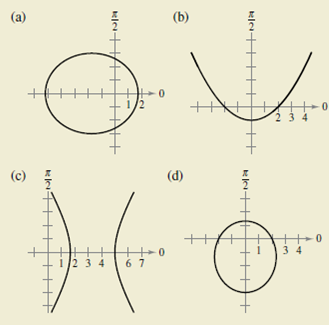Chapter 10.6, Problem 48E

Chapter
Section
Textbook Problem

HOW DO YOU SEE IT? Identify the conic in the graph and give the possible values for the eccentricity.(a)

To determine
The conic in the graph and find out its possible values of eccentricity.Explanation

The curve of the above graph is an ellipse.

The Length of major axis approximately is 2a=7.

The Length of minor axis approximately is 2b=6. So,

c=a2b2=(72)2(62)2=3

(b)

To determine
The conic in the graph and find out its possible values of eccentricity.(c)

To determine
the conic in the graph and find out its possible values of eccentricity.(d)

To determine
The conic in the graph and find out its possible values of eccentricity.Still sussing out bartleby?

Check out a sample textbook solution.

See a sample solution

The Solution to Your Study Problems

Bartleby provides explanations to thousands of textbook problems written by our experts, many with advanced degrees!

Get Started

Solve the equations in Exercises 112 for x (mentally, if possible). x1=cx+d(c1)

Finite Mathematics and Applied Calculus (MindTap Course List)

Draw, in standard position, the angle whose measure is given. 19. 34rad

Single Variable Calculus: Early Transcendentals, Volume I

Write the following numbers in word form. .0005712

Contemporary Mathematics for Business & Consumers